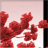# Move existing points within an existing featureclass in python

1862
0
11-24-2010 04:22 PMNew Contributor III
I'm wanting to move existing points within a featureclass (ArcGIS 10). I have an x and y field in the featureclass to where I want the point to be moved to. I've had a look in the online help and it explains how to insert a new point but not change the existing ones. The following code doesn't fall over but it also doesn't do anything. Am I going around this the correct way? Am I just missing something?

```    rows = arcpy.UpdateCursor(p_data2)
shapeName = arcpy.Describe(p_data2).shapeFieldName

for row in rows:
new_point_x = row.NEAR_X
new_point_y = row.NEAR_Y
old_point_x = row.POINT_X
old_point_y = row.POINT_Y
feat = row.getValue(shapeName)
pnt = feat.firstPoint
print "New Point %i %i" % (new_point_x,new_point_y)
print "Old Point %i %i" % (old_point_x,old_point_y)
print "Info %i %i" % (pnt.X,pnt.Y)
pnt.X = new_point_x
pnt.Y = new_point_y
print "Info %i %i" % (pnt.X,pnt.Y)
rows.updateRow(row)
```
Thanks

Mandy
Tags (5)
0 Replies